## How to Calculate and Solve for Water Activity | Ball Mill Sizing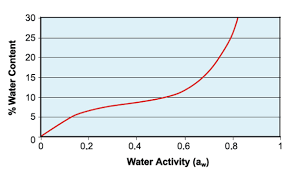The image above represents water activity.

To compute for water activity, two essential parameters are needed and these parameters are Partial Pressure of Water (Pw) and Equilibrium Vapour Pressure of Water at Same Temperature (V.P.w).

The formula for calculating water activity:

aw = Pw/V.P.w

Where:

aw = Water Activity | Drying
Pw = Partial Pressure of Water
V.P.w = Equilibrium Vapour Pressure of Water at Same Temperature

Let’s solve an example;
Find the water activity when the partial pressure of water is 22 and the equilibrium vapour pressure of water at same temperature is 28.

This implies that;

Pw = Partial Pressure of Water = 22
V.P.w = Equilibrium Vapour Pressure of Water at Same Temperature = 28

aw = Pw/V.P.w
aw = 22/28
aw = 0.78

Therefore, the water activity is 0.78.

Calculating the Partial Pressure of Water when the Water Activity and the Equilibrium Vapour Pressure of Water at Same Temperature is Given.

Pw = aw x V.P.w

Where;

Pw = Partial Pressure of Water
aw = Water Activity | Drying
V.P.w = Equilibrium Vapour Pressure of Water at Same Temperature

Let’s solve an example;
Find the partial pressure of water when the water activity is 24 and the equilibrium vapour pressure of water at same temperature is 14.

This implies that;

aw = Water Activity | Drying = 24
V.P.w = Equilibrium Vapour Pressure of Water at Same Temperature = 14

Pw = aw x V.P.w
Pw = 24 x 14
Pw = 336

Therefore, the partial pressure of water is 336.

## How to Calculate and Solve for r.p.m of Driver Pulley | Pulley Size Calculations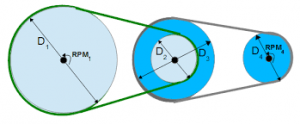The image above represents r.p.m of driver pulley.

To compute for r.p.m of driver pulley, three essential parameters are needed and these parameters are Diameter of Driven Pulley (Db), r.p.m. of Driven Pulley (rb) and Diameter of Driver Pulley (Db).

The formula for calculating r.p.m of driver pulley:

ra = (Db x rb/Da

Where:

ra = r.p.m. of Driver Pulley
Db = Diameter of Driven Pulley
rb = r.p.m. of Driven Pulley
Da = Diameter of Driver Pulley

Let’s solve an example;
Find the r.p.m of driver pulley when the diameter of driven pulley is 21, the r.p.m of driven pulley is 18 and the diameter of driver pulley is 14.

This implies that;

Db = Diameter of Driven Pulley = 21
rb = r.p.m. of Driven Pulley = 18
Da = Diameter of Driver Pulley = 14

ra = (Db x rb/Da
ra = (21 x 18)/14
ra = 378/14
ra = 27

Therefore, the r.p.m of driver pulley is 27 Hz.

## How to Calculate and Solve for r.p.m of Driven Pulley | Pulley Size Calculations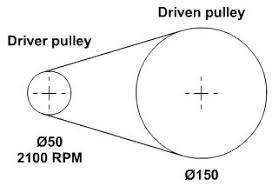The image above represents r.p.m of driven pulley.

To compute for r.p.m of driven pulley, three essential parameters are needed and these parameters are Diameter of Driven Pulley (Db), r.p.m. of Driver Pulley (ra) and Diameter of Driver Pulley (Db).

The formula for calculating r.p.m of driven pulley:

rb = (Da x ra/Db

Where:

rb = r.p.m. of Driven Pulley
Da = Diameter of Driver Pulley
ra = r.p.m. of Driver Pulley
Db = Diameter of Driven Pulley

Let’s solve an example;
Given that the diameter of driver pulley is 10, the r.p.m of driver pulley is 4 and the diameter of driven pulley is 6. Find the r.p.m of driven pulley?

This implies that;

Da = Diameter of Driver Pulley = 10
ra = r.p.m. of Driver Pulley = 4
Db = Diameter of Driven Pulley = 6

rb = (Da x ra/Db
rb = (10 x 4)/6
rb = 40/6
rb = 6.67

Therefore, the r.p.m of driven pulley is 6.67 Hz.

## How to Calculate and Solve for Diameter of Driver Pulley | Pulley Size Calculations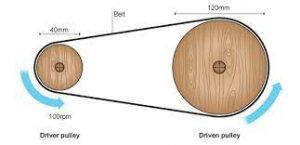The image above represents diameter of driver pulley.

To compute for diameter of driver pulley, three essential parameters are needed and these parameters are Diameter of Driven Pulley (Db), r.p.m. of Driver Pulley (ra) and r.p.m. of Driven Pulley (rb).

The formula for calculating diameter of driver pulley:

Da = (Db x rb/ra

Where:

Da = Diameter of Driver Pulley
Db = Diameter of Driven Pulley
rb = r.p.m. of Driven Pulley
ra = r.p.m. of Driver Pulley

Let’s solve an example;
Find the diameter of driver pulley when the diameter of driven pulley is 12, the r.p.m of driven pulley is 24 and the r.p.m of driver pulley is 10.

This implies that;

Db = Diameter of Driven Pulley = 12
rb = r.p.m. of Driven Pulley = 24
ra = r.p.m. of Driver Pulley = 10

Da = (Db x rb/ra
Da = (12 x 24)/10
Da = 288/10
Da = 28.8

Therefore, the diameter of driver pulley is 28.8 m.

## How to Calculate and Solve for Diameter of a Driven Pulley | Pulley Size Calculations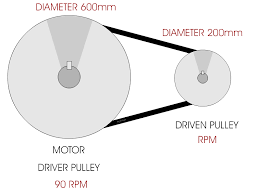The image above represents the diameter of a driven pulley.

To compute for diameter of a driven pulley, three essential parameters are needed and these parameters are Diameter of Driver Pulley (Da), r.p.m. of Driver Pulley (ra) and r.p.m. of Driven Pulley (rb).

The formula for calculating the diameter of a driven pulley:

Db = (Da x ra)/rb

Where:

Db = Diameter of Driven Pulley
Da = Diameter of Driver Pulley
ra = r.p.m. of Driver Pulley
rb = r.p.m. of Driven Pulley

Let’s solve an example;
Find the diameter of driven pulley when the diameter of driver is 3, the r.p.m of driver pulley is 8 and r.p.m of driven pulley is 22.

This implies that;

Da = Diameter of Driver Pulley = 3
ra = r.p.m. of Driver Pulley = 8
rb = r.p.m. of Driven Pulley = 22

Db = (Da x ra)/rb
Db = (3 x 8)/22
Db = 24/22
Db = 1.09

Therefore, the diameter of driven pulley is 3 m.

## How to Calculate and Solve for Shaft Length | Ball Mill Sizing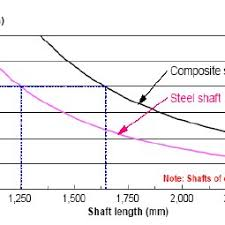The image above represents shaft length.

To compute for shaft length, four essential parameters are needed and these parameters are Applied Torque (T), Length of Shaft (L), Modulus of Rigidity (G) and Diameter of Shaft (D).

The formula for calculating shaft length:

θ = T x L x 32/G x π x D4

Where:

θ = Shaft Length
T = Applied Torque
L = Length of Shaft
G = Modulus of Rigidity
D = Diameter of Shaft

Let’s solve an example;
Find the shaft length when the applied torque is 8, the length of shaft is 12, the modulus of rigidity is 4 and the diameter of shaft is 10.

This implies that;

T = Applied Torque = 8
L = Length of Shaft = 12
G = Modulus of Rigidity = 4
D = Diameter of Shaft = 10

θ = T x L x 32/G x π x D4
θ = (8) x (12) x 32/(4) x π x (10)4
θ = 3072/(4) x π x (10000)
θ = 3072/125663.7
θ = 0.024

Therefore, the shaft length is 0.024 m.

Calculating the Applied Torque when the Shaft Length, the Length of Shaft, the Modulus of Rigidity and the Diameter of Shaft is Given.

T = θ x G x π x D4 / L x 32

Where;

T = Applied Torque
θ = Shaft Length
L = Length of Shaft
G = Modulus of Rigidity
D = Diameter of Shaft

Let’s solve an example;
Find the applied torque when the shaft length is 10, the length of shaft is 5, the modulus of rigidity is 4 and the diameter of shaft is 2.

This implies that;

θ = Shaft Length = 10
L = Length of Shaft = 5
G = Modulus of Rigidity = 4
D = Diameter of Shaft = 2

T = θ x G x π x D4 / L x 32
T = 10 x 4 x π x 24 / 5 x 32
T = 40 x π x 16 / 160
T = 2010.6 / 160
T = 12.56

Therefore, the applied torque is 12.56 m.

## How to Calculate and Solve for Shaft Diameter | Ball Mill Sizing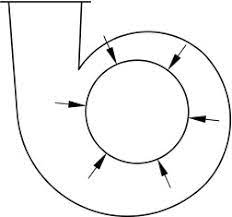The image above represents shaft diameter.

To compute for shaft diameter, two essential parameter are needed and these parameters are Shaft Power (P) and 75% of Critical Speed (N).

The formula for calculating shaft diameter:

D = ³√(1.33 x 106 x P/N)

Where:

D = Shaft Diameter
P = Shaft Power
N = 75% of Critical Speed

Let’s solve an example;
Find the shaft diameter when the shaft power is 28 and the 75% of critical speed is 7.

This implies that;

P = Shaft Power = 28
N = 75% of Critical Speed = 7

D = ³√(1.33 x 106 x P/N)
D = ³√(1.33 x 106 x 28/7)
D = ³√(37240000/7)
D = ³√(5320000)
D = 174.57

Therefore, the shaft diameter is 174.57 m.

Calculating the Shaft Power when the Shaft Diameter and the 75% of Critical Speed is Given.

P = D3 x N / 1.33 x 106

Where;

P = Shaft Power
D = Shaft Diameter
N = 75% of Critical Speed

Let’s solve an example;
Find the shaft power when the shaft diameter is 3 and the 75% of critical speed is 10.

This implies that;

D = Shaft Diameter = 3
N = 75% of Critical Speed = 10

P = D3 x N / 1.33 x 106
P = 33 x 10 / 1.33 x 106
P = 9 x 10 / 1.33 x 106
P = 90 / 140.98
P = 0.64

Therefore, the shaft power is 0.64 m/s.

## How to Calculate and Solve for Mill Speed | Ball Mill Sizing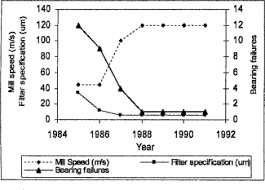The image above represents the mill speed.

To compute for mill speed, two essential parameters are needed and these parameters are Speed Percentage (N%) and Critical Speed (Nc).

The formula for calculating mill speed:

MS = N% x Nc

Where:

MS = Mill Speed
N% = Speed Percentage
Nc = Critical Speed

Let’s solve an example;
Find the mill speed when the speed percentage is 12 and the critical speed is 10.

This implies that;

N% = Speed Percentage = 12
Nc = Critical Speed = 10

MS = N% x Nc
MS = (12/100) x (10)
MS = (0.12) x (10)
MS = 1.2

Therefore, the mill speed is 1.2 m/s.

Calculating the Speed Percentage when the Mill Speed and the Critical Speed is Given.

N% = MS / Nc

Where;

N% = Speed Percentage
MS = Mill Speed
Nc = Critical Speed

Let’s solve an example;
Find the speed percentage when the mill speed is 24 and the critical speed is 6.

This implies that;

MS = Mill Speed = 24
Nc = Critical Speed = 6

N% = MS / Nc
N% = 24 / 6
N% = 3

Therefore. the speed percentage is 3 m/s.

## How to Calculate and Solve for Critical Mill of Speed | Ball Mill Sizing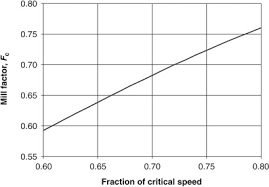The image above represents critical mill of speed.

To compute for critical mill of speed, two essential parameters are needed and these parameters are Mill Diameter (D) and Diameter of Balls (d).

The formula for calculating critical mill of speed:

Nc = 42.3/√(D – d)

Where:

Nc = Critical Speed of Mill
D = Mill Diameter
d = Diameter of Balls

Let’s solve an example;
Find the critical speed of mill when the mill diameter is 12 and the diameter of balls is 6.

This implies that;

D = Mill Diameter = 12
d = Diameter of Balls = 6

Nc = 42.3/√(D – d)
Nc = 42.3/√(12 – 6)
Nc = 42.3/√(6)
Nc = 42.3/2.44
Nc = 17.26

Therefore, the critical speed of mill is 17.26 m/s.

Calculating for Mill Diameter when the Critical Speed of Mill and the Diameter of Balls is Given.

D = (42.3 / Nc)2 + d

Where;

D = Mill Diameter
Nc = Critical Speed of Mill
d = Diameter of Balls

Let’s solve an example;
Find the mill diameter when the critical speed of mill is 20 and the diameter of bills is 10.

This implies that;

Nc = Critical Speed of Mill = 20
d = Diameter of Balls = 10

D = (42.3 / Nc)2 + d
D = (42.3 / 20)2 + 10
D = 4.47 + 10
D = 14.47

Therefore, the mill diameter is 14.47.

## How to Calculate and Solve for Shaft Power | Ball Mill Length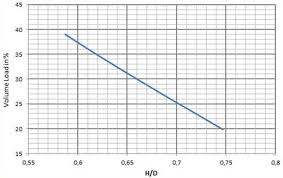The image above represents shaft power | ball mill length.

To compute for shaft power | ball mill length, six essential parameters are needed and these parameters are Value of C, Volume Load Percentage (J), % Critical Speed (Vcr), Bulk Density (s.g), Mill Length (L) and Mill Internal Diameter (D).

The formula for calculating shaft power | ball mill length:

P = 7.33 x C X J X Vcr x (1 – 0.937) x [1 – 0.1/29 – 10Vcr] x s.g. x L x D2.3

Where:

P = Shaft Power | Ball Mill Length
Vcr = % Critical Speed
sg = Bulk Density
L = Mill Length
D = Mill Internal Diameter

Let’s solve an example;
Find the shaft power | ball mill length when the volume load percentage is 8, the %critical speed is 10, the bulk density is 3. the mill length is 14 and the mill internal diameter is 16.

This implies that;

J = Volume Load Percentage = 8
Vcr = % Critical Speed = 10
sg = Bulk Density = 3
L = Mill Length = 14
D = Mill Internal Diameter = 16

P = 7.33 x C X J X Vcr x (1 – 0.937) x [1 – 0.1/29 – 10Vcr] x s.g. x L x D2.3
P = 7.33 x 1 x 8 x 10 x (1 – 0.937) x [1 – 0.1/29 – 10(10)] x 3 x 14 x 162.3
P = 36.94 x [1 – 0.1/2-91] x 3 x 14 x 588.133
P = 36.94 x [1 – 0.1/4.038e-28] x 24701.609
P = 36.94 x [1 – 2.47e+26] x 24701.609
P = 36.94 x -2.475e+26 x 24701.609
P = -2.259

Therefore, the shaft power | ball mill length is – 2.259 W.